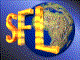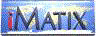| iMatix home page | << | < | > | >>SFLVersion 2.11

### tree_insert

```#include "sfltree.h"
int tree_insert (TREE **root, void *tree, TREE_COMPARE *comp,
Bool allow_duplicates)
```

#### Synopsis

Inserts a node into an existing tree. Initialises node pointers and colour to correct values. The data used by the compare functions must be filled in so that tree_insert can find the correct place in the tree to insert the node.

#### Source Code - (sfltree.c)

```{
TREE
*current,
*parent;
int
last_comp = 0;

/* find where node belongs */
current = *root;
parent  = NULL;
while (current != TREE_NULL)
{
parent  = current;
last_comp = (comp) (tree, current);
switch (last_comp)
{
case -1: current = current-> left;  break;
case  1: current = current-> right; break;
default: if (allow_duplicates)
current = current-> left;
else
return TREE_DUPLICATE;

}
}

/* setup new node */
((TREE *) tree)-> parent = parent;
((TREE *) tree)-> left   = TREE_NULL;
((TREE *) tree)-> right  = TREE_NULL;
((TREE *) tree)-> colour = RED;

/* insert node in tree */
if (parent)
switch (last_comp)
{
case  1: parent-> right = tree;  break;
default: parent-> left  = tree;
}
else
*root = tree;

insert_fixup (root, tree);
return (TREE_OK);
}
```

 | << | < | > | >>Copyright © 1996-2000 iMatix Corporation